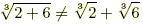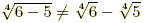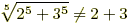Root Rules
nth Root Rules

Algebra rules for nth roots are listed below. Radicalexpressions can be rewritten using exponents, so the rules below are a subset of the exponent rules.

 For all of the following, n is an integer and n ≥ 2. Definitions1.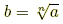if both b ≥ 0 and bn = a. Examples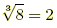because 23 = 8. 2. If n is odd then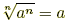.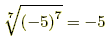3. If n is even then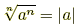.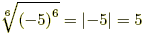4. If a ≥ 0 then.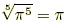and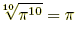Distributing (a ≥ 0 and b ≥ 0)1.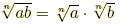2.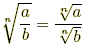(b ≠ 0) Examples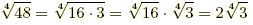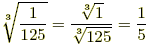3.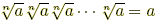(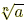multiplied by itself n times equals a)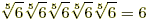4.(m ≥ 0)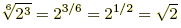Rationalizing the Denominator (a > 0, b > 0, c > 0) Examples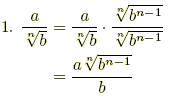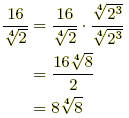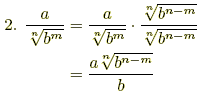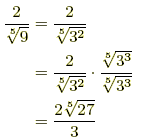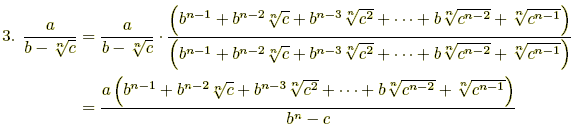Example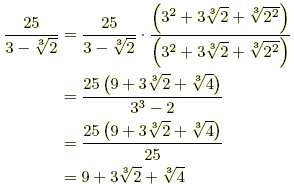Careful!!1.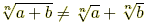2.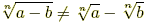3.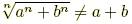Examples